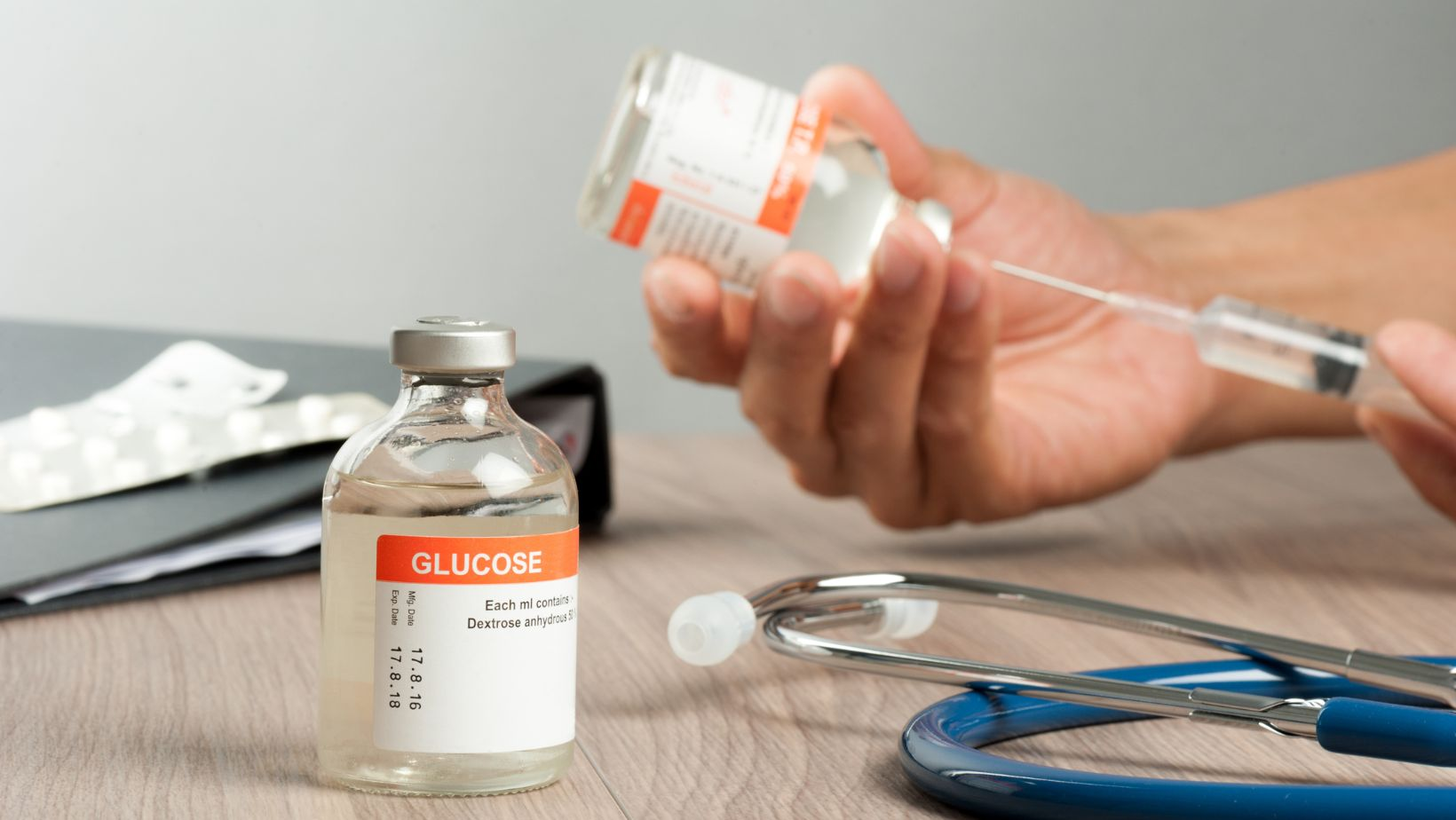# Accurate Calculation on How Many Grams of Glucose (C6H12O6) are Contained in 555 ml of a 1.77 m Glucose Solution?## How Many Grams of Glucose (C6H12O6) are Contained in 555 ml of a 1.77 m Glucose Solution?

If you’re wondering how many grams of glucose (C6H12O6) are present in a 555 ml solution with a concentration of 1.77 M, you’ve come to the right place. Let’s break it down and find out the answer.

To determine the number of grams of glucose in the solution, we need to consider its molarity and volume. The molarity (M) represents the number of moles of solute per liter of solution. In this case, our solution has a concentration of 1.77 M, meaning there are 1.77 moles of glucose per liter.

Next, we’ll calculate the number of moles in our given volume, which is 555 ml or 0.555 liters. By multiplying the molarity by the volume in liters (1.77 M * 0.555 L), we can find that there are approximately 0.98235 moles of glucose in our solution.

Finally, to convert moles to grams, we need to know the molecular weight of glucose (C6H12O6). By adding up the atomic masses for each element (6 carbon atoms with an atomic mass of 12 g/mol + 12 hydrogen atoms with an atomic mass of 1 g/mol + 6 oxygen atoms with an atomic mass of 16 g/mol), we get a total molecular weight for glucose as 180 g/mol.

Multiplying our calculated number of moles by the molecular weight (0.98235 * 180 g/mol), we find that there are approximately 176.82 grams of glucose present in our solution.

## Calculating the Number of Grams of Glucose

To determine the number of grams of glucose (C6H12O6) contained in 555 ml of a 1.77 M glucose solution, we need to follow a simple calculation process. Let’s break it down step by step:

1. Convert milliliters to liters:
• We have 555 ml, which is equivalent to 0.555 liters.
1. Use the molarity formula to calculate the number of moles:
• Molarity (M) is defined as moles of solute per liter of solution.
• Given that the glucose solution has a molarity of 1.77 M, we can calculate the number of moles using the formula: Moles = Molarity * Volume.
• Plugging in our values, we have: Moles = 1.77 mol/L * 0.555 L.
1. Calculate the grams using molecular weight:
• The molecular weight or molar mass of glucose (C6H12O6) is approximately 180 g/mol.
• To find the number of grams, multiply the number of moles by the molar mass: Grams = Moles * Molecular Weight.

Let’s summarize our calculations in a table:

 Given Information Volume (ml) 555 Volume (L) 0.555 Molarity (M) 1.77 Molecular Weight (g/mol) 180

 Calculations Moles \$1.77 times{0.555}\$ Grams \$ text{Moles} times{180}\$

After performing the calculations, we find that the number of grams of glucose in 555 ml of a 1.77 M glucose solution is approximately [177.885 grams](formatted: 177.885 grams).

Paige is a loving wife and an excellent chef. She owns Justalittlebite.com, a website that shares recipes and cooking tips. Paige loves spending time in the kitchen, where she can experiment with new flavors and techniques. Her husband appreciates her delicious cooking, as do her many friends and followers online. When she's not in the kitchen, Paige enjoys spending time with her family and friends.